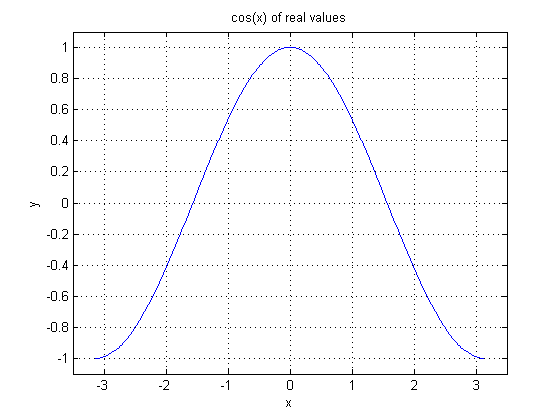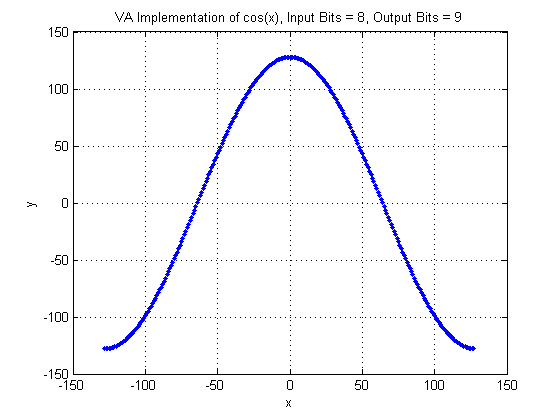## Operator COS

The operator calculates the cosine of the input.

The input range of the cosine function is [∞, ∞]. Because of the periodicity of the cosine function the input range of the VisualApplets operator is limited to [π, π[ i.e. the minimum value at the input is -π and the maximum value at the input plus 1 is π Thus, it it not possible to have the value +π at the input.

The argument x of the cosine function is therefore determined by

where

is the bit width at the input link.

The results of the cosine function are in the range [-1, 1]. The output value range of the operator in VisualApplets is mapped to

where

is the bit width at the output link. Thus the output value is

The following image shows the plot of the cosine function.In the next figure, the VisualApplets operator implementation is shown. Note the input and output bit widths.Le't have a look at an input pixel value. For example -50. With the given input bit width of 8, the real value representation of the pixel value is -50 * pi / 128. The cosine result will then be 0.33. In pixel value representation this result becomes 43 which is the same as shown in the plot.

### I/O Properties

Property Value
Operator Type O

Bit Width [8, 12] [8, 32]
Arithmetic signed signed
Parallelism any as I
Kernel Columns 1 as I
Kernel Rows 1 as I
Img Protocol {VALT_IMAGE2D, VALT_LINE1D, VALT_PIXEL0D} as I
Color Format VAF_GRAY as I
Color Flavor FL_NONE as I
Max. Img Width any as I
Max. Img Height any as I

None

### Examples of Use

The use of operator COS is shown in the following examples: